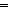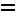## Synthesis and structures of β-diketiminatotin(ii) halides, an amide and of Sn(E)[{N(R)C(Ph)}2CH](NR2) (E = S or Se, R = SiMe3)

Author affiliations

### Abstract

Treatment of [Li(L1)]2 (1) or K(L2) (2) with SnX2 in Et2O yielded the heteroleptic β-diketiminatotin(II) halides Sn(L1)Cl (3a), Sn(L1)Br (3b) or Sn(L2)Cl (4), even when an excess of the alkali metal β-diketiminate was used [L1 = {N(R)C(Ph)}2CH, L2 = {N(R)C(Ph)CHC(But)N(R)}, R = SiMe3]. From 2 and half an equivalent each of SnCl2·2H2O and SnCl2, or one equivalent of SnCl2·2H2O, the product was Sn(L3)Cl (5) or Sn(L4)Cl (6), in which one or both of the N–R bonds of L1 had been hydrolytically cleaved; the compound Sn(L5)Cl (7) was similarly obtained from 1 and an equivalent portion of SnCl2·2H2O [L3 = {N(R)C(Ph)CHC(But)N(H)}, L4 = {N(H)C(Ph)CHC(But)N(H)} and L5 = {N(H)C(Ph)}2CH]. The halide exchange between 3a and 3b, studied by two-dimensional 119Sn{1H}-NMR spectroscopy, is attributed to implicate a (µ-Cl)(µ-Br)-dimeric intermediate or transition state. The 13C{1H}-NMR spectra of 3a or 3b showed two distinct resonances for each group, which coalesced on heating, corresponding to ΔG338 K = 69.4 (3a) or 72.8 (3b) kJ mol−1. The chloride ligand of 3a was readily displaced by treatment with NaNR2, CF3SO3H or CH2(COPh)2, yielding Sn(L1)X [X = NR2 (8), O3SCF3 (9) or {OC(Ph)}2CH (10)]. Oxidative addition of sulfur or selenium to 8 gave the tin(IV) terminal chalcogenides Sn(E)(L1)(NR2) [E = S (11) or Se (12)]. The X-ray structures of the cocrystal of 3a/3b and of the crystalline compounds 5, 6, 8, and 11 are presented, as well as multinuclear NMR spectra of each of the new compounds.## Article information

Submitted
18 Aug 2004
Accepted
20 Oct 2004
First published
16 Nov 2004

Dalton Trans., 2004, 4193-4201
Article type
Paper
Permissions### Synthesis and structures of β-diketiminatotin(II) halides, an amide and of Sn(E)[{N(R)C(Ph)}2CH](NR2) (E = S or Se, R = SiMe3)

P. B. Hitchcock, J. Hu, M. F. Lappert and J. R. Severn, Dalton Trans., 2004, 4193
DOI: 10.1039/B412765JSocial activity